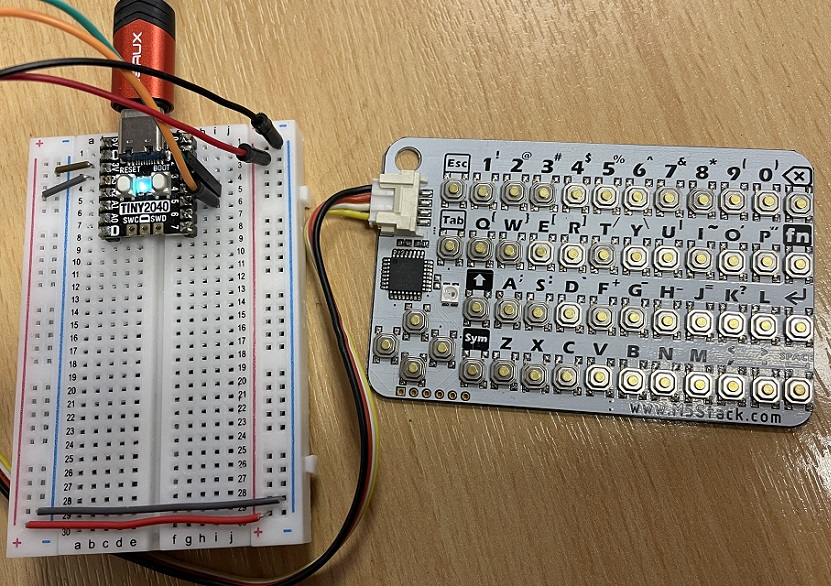# Raspberry Pi Pico CardKB Mini Keyboard

The CarbKB Mini Keyboard is a credit card sized board with pushbuttons arranged into a full keyboard. It has a microcontroller on the board itself which handles all of the button presses and makes them available over i2c. Search enough on the WWW and you can find a UK supplier that will let you have this for under £10.

The photograph shows how I hooked this up to my Tiny 2040. I had a grove to dupont adapter and connected the power pins to GND and 3V3. The other two pins are the SDA and SCL pins. I connected these to GP4 and GP5 on the Pico. The power pins are colour coded. The other two can be swapped around if you can't get your Pico to see the device.In this first program I just wanted to be able to get a chunk of text entered. I was looking for a whole message rather than just a single character. I also wanted to be able to see the text as I typed it. The code here just gets you to type in some text and press enter. The text you type is printed out at the end.

```from machine import Pin, I2C
from time import sleep

i2c = I2C(0,sda=Pin(4), scl = Pin(5))

def get_message():
message = ""
data = -1
while data!=13:
if data!=0:
message += chr(data)
sleep(0.1)
return message

def get_message1():
message = ""
data = -1
while data!=13:
if data!=0:
message += chr(data)
print(chr(data), end="")
sleep(0.1)
print()
return message

while True:
msg = get_message1()
print(msg)
sleep(0.1)
```

I then thought about the occasions where I might want to have strictly numeric information being entered. The following program has code for both integers and floats. Change the code at the very end if you want to test it for integers rather than floats.

```from machine import Pin, I2C
from time import sleep

i2c = I2C(0,sda=Pin(4), scl = Pin(5))

def get_message():
message = ""
data = -1
while data!=13:
if data!=0:
message += chr(data)
sleep(0.1)
return message

def get_message1():
message = ""
data = -1
while data!=13:
if data!=0:
message += chr(data)
print(chr(data), end="")
sleep(0.1)
print()
return message

def get_integer(prompt):
print(prompt, end="")
message = ""
data = -1
while data!=13:
if data!=0 and chr(data) in "0123456789":
message += chr(data)
print(chr(data), end="")
sleep(0.1)
print()
return int(message)

def is_float(n):
try:
x = float(n)
except:
return False
else:
return True

def get_float(prompt):
print(prompt, end="")
message = ""
data = -1
while data!=13:
if data!=0 and chr(data) in "0123456789.":
if is_float(message + chr(data)):
message += chr(data)
print(chr(data), end="")
sleep(0.1)
print()
return float(message)

while True:
msg = get_float("What's the answer? ")
print(msg)
sleep(0.1)
```

Finally, I put together a program to make a times table quiz.

```from machine import Pin, I2C
from time import sleep
from random import randint

i2c = I2C(0,sda=Pin(4), scl = Pin(5))

def get_integer(prompt):
print(prompt, end="")
message = ""
data = -1
while data!=13:
if data!=0 and chr(data) in "0123456789":
message += chr(data)
print(chr(data), end="")
sleep(0.1)
print()
return int(message)

score = 0
result = 0
a = 0
b = 0

for i in range(12):
a = randint(2,12)
b = randint(2,12)
result = a * b
question = "What is " + str(a) + " x " + str(b) + "? "